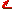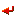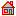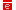Unify materials: spreadsheets and modelling

This page contains material to support the use of spreadsheets for modelling - with examples drawn mainly from chemistry.

Introduction

Most of the examples used here were devised to help 'A' level pupils and those in the first year at university to understand aspects of chemical equilibrium. They have been used in a number of European countries. A brief explanation of their uses can be downloaded here and a paper produced by an ERASMUS/SOCRATES project which explains them in more detail can be found here. The first of these downloads is from the book 'Learning with artificial worlds: computer-based modelling across the curriculum' edited by Harvey Mellar et al. (Institute library code Loz Butb MEL) which is an excellent resource for those who wish to understand this topic better. A paper giving some examples from physics can be found here.Return to top of this page

Terminology

Essentially there are two ways in which learners can use spreadsheets for modelling activities.

1. they can write their own models - the 'expressive' mode of use

2. they can examine the models produced by others - the 'exploratory' mode of use.

Usually 'exploratory' modelling involves running a simulation - a model written by an expert - although this need not be the case. One useful activity is to get learners to produce their own models and then to explore each others.Return to top of this page

Expressive example

A (technically) simple modelling activity that was devised for use with people studying 'A' level chemistry can be downloaded here. If you need help understanding the chemistry of this example - or just want more information on it - please see the section 'Addressing the Students' Difficulties' on page 2 of this article.) This example has been incorporated into both Nuffield and Salter's 'A' level chemistry courses to help students understand the nature of the equilibrium law and, by replacing boring repetitive but simple calculations, allows the learners to concentrate on the science rather than the maths.Return to top of this page

Exploratory examples

An example which allows learners to explore the effect of changing concentrations and temperatures on both the position of chemical equilibrium and the equilibrium concentrations of the various reactants can be found here. The calculations involved are both difficult to understand and very tedious to do, yet the chemical ideas illustrated are both very important and often misunderstood. Automating the calculations allows learners to concentrate on getting a 'feel' for what is happening - increasing their qualitative understanding of the process - which, contrary to most people's expectations, is often the hardest thing for them to get. This is an example of a 'micro-world' - a computer-based world which pupils can explore.

Other examples in this section illustrate the use of different kinds of macros.

The first uses a command macro to run a simple Monte-Carlo simulation of the chemical reaction between 'a' and 'b'. The user sets the initial amounts of a and b together with the probabilities of a molecule of a changing to b and of b changing to a. The system then selects molecules at random and 'throws a dice' to decide whether they should change or not. Over time, the ratio a/b moves to be the same as that of the two probabilities - irrespective of the initial amounts of a and b present. The macro and the 'display' sheet have been put in different workbooks - you will need to download both (preferably the macro first). Please feel free to put them together on your machine.

The second example uses a function macro to calculate the pH of a solution as a weak base is added to a weak acid. (Load the macro before the worksheet.) The programme is general - the user selects all the values for the 'Initial Data' box. In this case, as there are three competing equilibria in the solution, calculating the concentration of Hydrogen ions involves solving a quartic equation - and quartic equations have no analytic solution. The function macro solves the equation using the Newton-Raphson iterative method. This would be beyond the capabilities (and inclination) of virtually all 'A' level students yet this method allows them to compare the results of a 'real' titration with that given by theory - an interesting and important concept.Return to top of this page

Other examples

As was noted above, calculations are often a barrier to understanding in science. These barriers are of two sorts. The most obvious is where the calculations are difficult, but also just doing the same simple sum time and time again moves the learners' attention away from the concepts they are studying to the mechanics of the maths task.

Three examples where a spreadsheet has been used to address this latter problem are given below.

The first was used in the Salter's 'A' level to help pupils calculate energy changes in reactions. It can be downloaded here. The idea was to get pupils to work out which was the 'best' fuel for various purposes and by automating the calculations involved, the spreadsheet allows them to concentrate on the chemical ideas.

The second has been used with younger (KS4) pupils to help them interpret the results of an experiment designed to calculate the formula of magnesium oxide. It can be found here.

The final example has been used with 6th form pupils - but is really a 'fun' exercise designed to show how systems with feedback can move to chaotic (but deterministic) behaviour. It was used to illustrate the difference between 'exactness' and 'precision' - a distinction pupils often have trouble appreciating. It can be found here.Return to top of this page

Manuals

There are numerous manuals and tutorials available on the web to help you learn how to use spreadsheets - although almost all of these refer only to Excel.

Some of these are listed in the table below which contains three kinds of documents: manuals from Happy Computers, Kings College Canterbury and King Edward's School Birmingham ; three examples of worksheets written by Microsoft; and for more advanced users, an example and explanation of how to use pivot tables written for the IT PGCE at the Institute of Education (this analyses beer sales in a pub).

The table indicates whether the link is to a web page or a downloadable document, and in the latter case, the kind of file the document is. Because most of these downloadable files are large, some of them have been 'zipped' to reduce their size. To read these, first download them to your own machine and then double click on them and they will 'expand' themselves. You will then have a copy of the manual on your disc.

 Happy Computer's Excel 97 (.zip) Happy Computer's Excel 2000 (.zip) King Edward's Excel 97 (.zip) Canterbury's Excel 2000 (.pdf) Pivot table data (.xls) MS Excel lesson (.doc) MS Excel lesson (web) MS 'Analysing data' workshop (web)Return to top of this pageBack to Unify pre-visit site indexBack to Science and Technology Home PageBack to Institute of Education Home Page